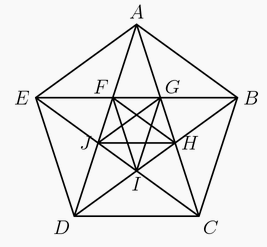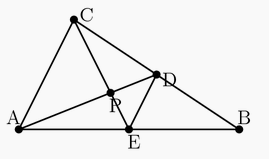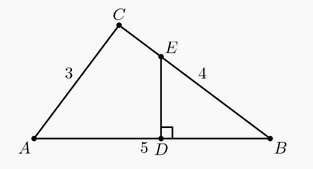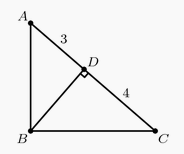###### back to index | new

Triangle $ABC$ has positive integer side lengths with $AB=AC$. Let $I$ be the intersection of the bisectors of $\angle B$ and $\angle C$. Suppose $BI=8$. Find the smallest possible perimeter of $\triangle ABC$.

Isosceles triangles $T$ and $T'$ are not congruent but have the same area and the same perimeter. The sides of $T$ have lengths $5$, $5$, and $8$, while those of $T'$ have lengths $a$, $a$, and $b$. Which of the following numbers is closest to $b$?

In $\triangle ABC$, $\angle C = 90^\circ$ and $AB = 12$. Squares $ABXY$ and $ACWZ$ are constructed outside of the triangle. The points $X$, $Y$, $Z$, and $W$ lie on a circle. What is the perimeter of the triangle?

What is the greatest possible perimeter of an isosceles triangle with sides of length $5x + 20$, $3x + 76$ and $x + 196$?

What is the radius of a circle inscribed in a triangle with sides of length $5$, $12$ and $13$ units?

The angles in a particular triangle are in arithmetic progression, and the side lengths are $4,5,x$. The sum of the possible values of x equals $a+\sqrt{b}+\sqrt{c}$ where $a, b$, and $c$ are positive integers. What is $a+b+c$?

Triangle $ABC$ has side-lengths $AB = 12, BC = 24,$ and $AC = 18.$ The line through the incenter of $\triangle ABC$ parallel to $\overline{BC}$ intersects $\overline{AB}$ at $M$ and $\overline{AC}$ at $N.$ What is the perimeter of $\triangle AMN?$

Triangle $ABC$ has $AB = 13$ and $AC = 15$, and the altitude to $\overline{BC}$ has length $12$. What is the sum of the two possible values of $BC$?

Bill walks $\tfrac12$ mile south, then $\tfrac34$ mile east, and finally $\tfrac12$ mile south. How many miles is he, in a direct line, from his starting point?

Two angles of an isosceles triangle measure $70^\circ$ and $x^\circ$. What is the sum of the three possible values of $x$?

The lengths of the sides of a triangle in inches are three consecutive integers. The length of the shortest side is $30\%$ of the perimeter. What is the length of the longest side?

The line $12x+5y=60$ forms a triangle with the coordinate axes. What is the sum of the lengths of the altitudes of this triangle?

In the figure shown below, $ABCDE$ is a regular pentagon and $AG=1$. What is $FG + JH + CD$?The two legs of a right triangle, which are altitudes, have lengths $2\sqrt3$ and $6$. How long is the third altitude of the triangle?

The $y$-intercepts, $P$ and $Q$, of two perpendicular lines intersecting at the point $A(6,8)$ have a sum of zero. What is the area of $\triangle APQ$?

Two sides of a triangle have lengths $10$ and $15$. The length of the altitude to the third side is the average of the lengths of the altitudes to the two given sides. How long is the third side?

In $\triangle ABC$, $AB = 86$, and $AC=97$. A circle with center $A$ and radius $AB$ intersects $\overline{BC}$ at points $B$ and $X$. Moreover $\overline{BX}$ and $\overline{CX}$ have integer lengths. What is $BC$?

In triangle $ABC$, medians $AD$ and $CE$ intersect at $P$, $PE=1.5$, $PD=2$, and $DE=2.5$. What is the area of $AEDC$?The area of $\triangle$$EBD is one third of the area of 3-4-5 \triangle$$ABC$. Segment $DE$ is perpendicular to segment $AB$. What is $BD$?Nondegenerate $\triangle ABC$ has integer side lengths, $\overline{BD}$ is an angle bisector, $AD = 3$, and $DC=8$. What is the smallest possible value of the perimeter?

Triangle $ABC$ has a right angle at $B$. Point $D$ is the foot of the altitude from $B$, $AD=3$, and $DC=4$. What is the area of $\triangle ABC$?A flagpole is originally $5$ meters tall. A hurricane snaps the flagpole at a point $x$ meters above the ground so that the upper part, still attached to the stump, touches the ground $1$ meter away from the base. What is $x$?

A right triangle has perimeter $32$ and area $20$. What is the length of its hypotenuse?

A triangle with side lengths in the ratio $3 : 4 : 5$ is inscribed in a circle with radius 3. What is the area of the triangle?

Point $P$ is inside equilateral $\triangle ABC$. Points $Q$, $R$, and $S$ are the feet of the perpendiculars from $P$ to $\overline{AB}$, $\overline{BC}$, and $\overline{CA}$, respectively. Given that $PQ=1$, $PR=2$, and $PS=3$, what is $AB$?